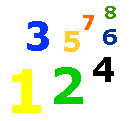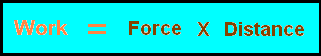# 4-13 Concept of Work

 Work is defined as how much force on an object times how much distance the object moves.  In physics, work is only being done when an object is moved a distance by a force.  The farther an object is moved, the more work that is being done.  The formula for work is:Let's say we move a 20 pound object 40 feet.  The work done would be 20 X 40 = 800.  Work is defined as foot-pounds.  The work done would be 800 foot-pounds of work.  Scientist also use "erg" and "joule."  A joule is about .738 foot-pounds. Complete the questions below.   Work is defined as how much on an object times how much an object moves. Scientist use a , about .738 foot-pounds to measure work. The an object is moved, the more work that is done. If an object, that weights 30 pounds, is moved 30 feet, then  foot-pounds of work will be done. If an object, that weighs 100 pounds, is move 1 foot, then foot-pounds of work will be done.